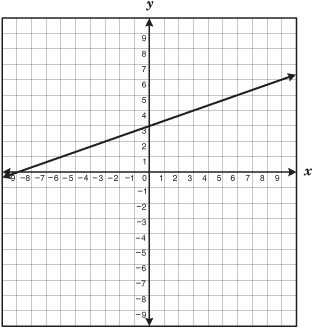# Comparing Functions

Directions: Using the digits 0-9, at most one time each, create five ordered pair that represent a linear function that has a greater rate of change than the following:(___,___) (___,___) (___,___) (___,___) (___,___)

How many different ones are there?

### Hint

What information tells you about the rate of change?

4, they are:
f(x)= {(0,1),(2,3),(4,5),(6,7),(8,9)}
f(x)= {(1,0),(3,2),(5,4),(7,6),(9,8)}
f(x)= {(0,5),(1,6),(2,7),(3,8),(4,9)}
f(x)= {(5,0),(6,1),(7,2),(8,3),(9,4)}

Source: Bryan Anderson

## Exponential Powers

Directions: Using the digits 0 to 9 at most one time each, place a digit …

1.I love this task! It really gets as the question of what rate of change is and comparing rates of change.

Question: Does a correct solution need to be able to use all 10 numbers? If we are only asked to find 4 ordered pairs, couldn’t (1,2) (3,4) (5,6) (7,8) also fit? Sure, the y-intercept does not fit the parameters of the task, as it would be (0,1), but since we are only asked to find 4 ordered pairs, could this also be a solution?

Thank you for all that you do and all of your thinking to come up with such great tasks!

•Sorry Nicole, that was a typo. If you look at the solution set, I listed 5 ordered pairs. I will correct the mistake.

(although I would say yes, using only 4 points the outline was to not use any number twice- disregarding other points that lie on the line. Using only 4 points should open up the number of solutions as well.)

2.I don’t understand how to solve this problem. Could you please explain? Can you use more than one line with the same slope?

•I don’t think so, lines with the same slope needs the same digit.